Thank you for visiting nature.com. You are using a browser version with limited support for CSS. To obtain the best experience, we recommend you use a more up to date browser (or turn off compatibility mode in Internet Explorer). In the meantime, to ensure continued support, we are displaying the site without styles and JavaScript.

# Si:P as a laboratory analogue for hydrogen on high magnetic field white dwarf stars

## Abstract

Laboratory spectroscopy of atomic hydrogen in a magnetic flux density of 105 T (1 gigagauss), the maximum observed on high-field magnetic white dwarfs, is impossible because practically available fields are about a thousand times less. In this regime, the cyclotron and binding energies become equal. Here we demonstrate Lyman series spectra for phosphorus impurities in silicon up to the equivalent field, which is scaled to 32.8 T by the effective mass and dielectric constant. The spectra reproduce the high-field theory for free hydrogen, with quadratic Zeeman splitting and strong mixing of spherical harmonics. They show the way for experiments on He and H2 analogues, and for investigation of He2, a bound molecule predicted under extreme field conditions.

## Introduction

The response of atoms to high magnetic fields has been of interest for many decades1,2,3,4. It has been suggested that magnetic flux densities up to 105 T (1 gigagauss) exist on the surface of white dwarf and cataclysmic variable stars5,6,7. This inference is based on a complex process that involves a combination of modelling the pattern of flux around the surface and up through the atmosphere of the star, and a search for transitions that are stationary with magnetic field2,3,4. These models are then compared with astrophysical spectra. Definitively extracting a magnetic field value from the comparison is challenging because the measured spectra are noisy with many overlapping features, as can be seen from some examples in Fig. 1. In some cases, predicted features are missing and in others there are extra features. Such discrepancies are usually attributed to the inhomogeneity of the magnetic field across the surface or line-of-sight, and possibly species other than hydrogen. At the same time, there is controversy about how such high fields could even persist for any significant length of time8, and the dearth of high-field magnetic white dwarfs (HFMWDs) found in binary systems9. Identification of the observed spectral features is further hindered at these fields because the calculation of theoretical spectra where the magnetic cyclotron energy (ħeB/me) is comparable to the coulombic binding energy (the Rydberg R=mee4/2κ2ħ2, where κ=4πɛ0) is non-trivial, especially for atomic species other than hydrogen, such as helium10,11,12. The difficulty is that in the regime of interest (B~B0=me2e3/2κ2ħ3) the Zeeman effect becomes quadratic13,14,15, the spherical symmetry of the Coulomb potential competes with the cylindrical symmetry of the magnetic field, and the Schrodinger equation is non-integrable, so producing quantum chaos16,17. The theory for the very complex Zeeman structure in atoms has been compared with laboratory absorption spectra in magnetic fields up to 8 T (refs 13, 14, 15). Although in Rydberg atoms the cyclotron energy can be more easily made equivalent to the substantially lower binding energy (the characteristic field is lowered by a factor n4 where n is the principal quantum number)18, the regime near B0 is receiving renewed interest because it has recently been shown that a new kind of magnetic chemical bond can be produced that supersedes the electronic bond19, creating new molecules such as He2.

The Hamiltonian for an electron in atomic hydrogen usually applied is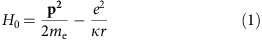where p=−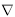is the momentum operator. When a hydrogen atom is subjected to a magnetic field, we replace p2 in equation 1 by (p+A)2, where A is the vector potential defined by B=× A. We now have, in units such that me=e=κ=ħ=1,where H0 is the zero-field Hamiltonian (equation 1), the second term, H1, is linear in field and the last term, H2, is responsible for the quadratic Zeeman effect. The Hamiltonian of equations 1 and 2 ignores spin orbit coupling and assumes the heavy ion mass limit, that is, it ignores the motion of the ion/donor. The general case is considered in refs 20,21. In other species, different approximations are necessary. Given the above questions, and the renewed interest in the high-field regime, it is crucial to validate experimentally the theory for the absorption spectra on which the inferred fields are based. Doing so directly for hydrogen is impractical because the required fields are two to three orders of magnitude beyond what is currently available in any laboratory22,23. Such tests become even more essential where atomic species beyond hydrogen are being considered, for example, helium10,11,12 or molecular hydrogen24,25,26,27 as for these cases the multiple particle calculations required are complex and involve approximations (for example, basis-state choices and sizes) that are not a priori guaranteed.

In low field, the Zeeman spectrum may be found from perturbation theory, the orbital and magnetic quantum numbers are constants of the motion. In this regime, B«B0 (half an atomic unit of field) the quadratic term is negligible, and the transitions shift linearly with field because H1LzBz produces the usual linear Zeeman energy ½mB (where Lz is the component of angular momentum along B||z and m is the magnetic quantum number). At very high field, the Coulomb potential is the perturbation and again there are constants of motion, but in the intermediate regime close to B0, the Zeeman spectrum is much more complex (Fig. 1).

It has long been known1 that shallow impurities in semiconductors exhibit energy level spectra that bear striking similarities to those of free atoms, and the canonical example is the Group V donor such as phosphorus in silicon (Si:P). In this case, the Group IV silicon atoms are tetrahedrally bonded, so the donor has one unused valence electron, loosely bound, which orbits about the ion core. The Si:P system yields well-resolved hydrogenic Lyman series spectra at zero field28,29 with excitations that are long lived and useful as alternatives to free atoms for quantum information applications30,31,32. The cyclotron energy and the donor-binding energy are equal for magnetic fields at around 30 T because the cyclotron and binding energies are scaled by the effective mass and dielectric constant1,33. Equation 2 still holds, and we now use appropriately scaled units so that m*=e=ɛrκ=ħ=1, see Table 1.

There are other possible analogues such as acceptors but these are much more complicated34, while other host materials such as III–V semiconductors generally produce such a small binding energy, or such wide homogeneous line widths, that the Rydberg states are not well resolved until B»B0 (refs 33,35), which thus sheds little light on the understanding of HFMWDs. To validate any computational scheme for helium in the high magnetic field regime accurately, a suitable analogue to measure would be the double donor sulphur in Si (ref. 36). Similarly, the ability to position phosphorous donors with atomic precision allows one to build coupled donor pairs to mimic molecular hydrogen24,25,26,27.

In this work, we use Si:P as an analogue of hydrogen in conditions similar to those at the surface of HFMWDs. The measured Lyman series spectra reproduce the theoretical predictions for free hydrogen under high field, with quadratic Zeeman splitting and strong mixing of spherical harmonics, demonstrating the possibility of the use of Si:P for modelling astrophysical data in the laboratory.

## Results

### High-field Lyman spectroscopy of Si:P

Figure 2 shows the measured and computed Lyman series spectra for silicon doped with phosphorus as a function of applied magnetic field (see Supplementary Data 1 for the raw data). The  cubic crystal axis, the field and the light propagation direction were chosen to be all parallel (Faraday geometry). The circular polarization dependence of the lowest two transitions is also shown in Fig. 2, for forward and reverse fields (Supplementary Data 2), showing that some transitions select one circular polarization. The experimental donor transitions are sharp and clearly resolved up to principal quantum number n=6.

### Theoretical field dependence of Si:P and free hydrogen

Figure 1 shows the positions and dipole transition strengths of the Balmer series for known HFMWDs, and Fig. 3 the Lyman series. Whereas the low field limit of the Balmer series has only three components that split linearly, at high field, the Hα (2p-3d) line splits into 3 × 5=15 components and so on. The quadratic Zeeman effect produces transitions that are forbidden at low field and a very complex Balmer spectrum (Fig. 1). The complexity is only somewhat reduced in the Lyman series (Fig. 3). Figure 2 shows the theoretical field dependence of the Si:P transition energies. The absolute level positions were calculated in atomic units, and these were converted to transition energies using a field-independent ground state energy of −2.277 R (see below). The magnetic field and transition energies were converted to tesla and meV using standard parameters (Table 1), so no fitting was performed.

The experimental and theoretical results are compared in Fig. 2 and Table 2. The calculation is in excellent agreement with the donor experiment, in both energy and polarization. There is also a striking correspondence between the calculated donor Lyman series (Fig. 2) and that for hydrogen (Fig. 3), especially between the circularly polarized transitions shown in red and blue. Figure 4 shows the very strong field-induced mixing, evident from the completely different wavefunction shapes at high field. At n=6, the states have a radius of 36 Bohr radii, or 114 nm, which corresponds to the average spacing between the donors (110 nm for the sample used) so that adjacent wavefunctions overlap, leading to broadening of the transitions. These wavefunctions are among the largest in radial extent ever investigated in the solid state, and for a free hydrogen atom to have a similar radius would require n~45.

### Influence of anisotropic crystal environment in Si:P

There are also some notable differences between Figs 2 and 3. The donors have a number of additional transitions, shown in cyan, that at first sight do not correspond with hydrogen, some transitions that ought to be forbidden for light incident along the field axis (the linearly polarized hydrogen transitions on Fig. 3, shown in green, such as the 2p0 are forbidden for Faraday geometry), and anti-crossings in these transitions. These effects all arise from the fact that while the hydrogen Hamiltonian is spherically symmetric, the donor electron experiences the periodic environment of the cubic crystal host. The constant energy surface in momentum space for electrons in vacuum is a sphere centred at the origin, whereas in silicon there are six equivalent conduction band valleys (Fig. 2d) (electrons with zero group-velocity have non-zero phase velocity—an effect of the periodic medium). The valleys are ellipsoidal, that is, anisotropic, and characterized by γ=mt/ml where mt and ml are the transverse and longitudinal effective masses. As a result of the donor anisotropy (γ≠1), some degeneracies are lifted at zero field, so that, for example, the 2p0 state is unpolarized and at lower energy than the 2p±.

Anisotropy produces an additional degree of freedom; the angle between the magnetic field and the valley axis. For free hydrogen atoms with spherical symmetry, we are at liberty to choose to quantize the angular momentum along the magnetic field, that is, B=(0,0,Bz). If the silicon sample is mounted with the field in the  crystallographic direction then two of the valleys (valleys 1 and 2 in Fig. 2d) have B||z, where z is the valley axis direction, whereas the other four have Bz. Anisotropy is the origin, for example, of the transition in Fig. 2 that starts at 39.2 meV at zero field but shows no linear Zeeman shift—it is the 2p±x for B||x (the subscript x on the level designation indicates the valley orientation).

### Ground state central cell correction and field dependence

The central unit cell of the silicon crystal where the donor atom sits has some strain and rearrangements of the electron cloud that break the host periodicity. These effects are strongly localized and do not extend more than a unit cell. Their net effect is to cause a short-range change in the potential additional to the hydrogenic Coulomb potential, which only affects states with significant amplitude at the centre, that is, donor states that have s-orbital character. In our Lyman series absorption experiment, this quantum defect only affects the ground 1s state. The observed transition energies (Fig. 2) are all larger than 1 Rydberg, that is, the extra potential is attractive.

This observation implies that this wavefunction has a smaller extent than predicted by scaling from hydrogen, which reduces the already small tuning with field (because H2A2 scales with r2). We therefore took the 1s state to be field independent.

We anticipate that the main improvements that can be made to the theory include small adjustments in the parameters, and a better calculation of the ground state and its field dependence, which must include proper treatment of the effects of the central cell correction.

### Quadratic versus anisotropic linear Zeeman effect

Because we have used much higher field and higher quality samples than previous donor spectroscopy33,37,38, we have also resolved new anti-crossings separately induced by either the linear or quadratic Zeeman couplings for the same low quantum number states important in white dwarf spectra (Fig. 1). These anti-crossings are very sensitive tests for the theory, and allow direct experimental measurement of the matrix elements of the Zeeman interaction. For the crystal orientation examined here (that is, ), there are examples of anti-crossings that are purely due to the same quadratic Zeeman coupling observed for free hydrogen, while others are purely due to the linear Zeeman coupling introduced by the anisotropy.

Looking at the experimental data of Fig. 2, we can clearly see the anti-crossing of the 2p±x line and the 3p0x line at 14 T, with splitting 1 meV. As the dominant basis-state components in the wavefunction are p± and p0, respectively, the term in the Hamiltonian responsible for the anti-crossing clearly couples ΔL=0 and Δm=±1 (where L is the orbital quantum number), and such terms are not present for hydrogen—it is only produced by the linear Zeeman effect in the presence of anisotropy. Anti-crossings due to this same linear Zeeman coupling may be observed for 2p±x and 3p±x with 4p0x (with much smaller radial overlap and hence smaller splitting). The downwards shift of 2p0x above 15 T results from similar coupling with 2p±x (with large radial overlap).

Turning to the quadratic Zeeman Hamiltonian, we observe anti-crossings with Δm=0 and ΔL=±2 that are entirely due to this coupling, and the same terms are present both in free hydrogen and the silicon donor with B||z. An example is evident in the p series (red lines on Figs 2 and 3), in which transitions gain and lose strength as a function of field. The p series is coupled by the quadratic Zeeman interaction to the f (and h and so on) series, and whereas Lyman transitions from 1s to p are dipole allowed, transitions to f are forbidden. The f states have the stronger field dependence and the 4f cuts through the p series leaving gaps. Figure 5 (Supplementary Data 3) shows a detail of Fig. 2 around the anti-crossing of the 4f with the 5p.

## Discussion

Having successfully verified the theory of hydrogen in high magnetic fields, we can return to the consideration of the white dwarf star spectra in Fig. 1. The centre panel shows the calculated hydrogen spectrum at each magnetic field with the dipole strength of each transition reflected in the width of the line. For the six magnetic white dwarfs shown, we have selected the magnetic field that best fits the measured spectrum. There is a good degree of agreement for all spectra and where extra or missing features are present, we can be confident that other effects are responsible (such as, for example, other species or strongly inhomogeneous magnetic fields).

In summary, we have experimentally investigated the spectroscopy of a solid-state analogue of hydrogen in conditions equivalent to the surface of the highest field magnetic white dwarf known. The calculated state energies, their anti-crossings, and the selection rules show excellent agreement with experiment up to the highest fields, thus validating the theoretical approach and providing confidence in its use for modelling astrophysical data. The simple scaling between the binding energies for Si:P and hydrogen is extremely well known, and here we show that much more subtle use can be made of it for measurement of Zeeman couplings. These quadratic Zeeman couplings and anti-crossings are responsible for the complexity of the predicted field dependence of the Balmer spectrum (Fig. 1), and although the effect of the quadratic Zeeman interaction has been detected in both free atoms1,15 and solids28,29,33,34,35,37,38, its characteristic anti-crossings with Δm=0 and ΔL=±2 have not been observed previously (and neither have the purely linear Zeeman, Bz anisotropy induced, anti-crossings with Δm=±1 and ΔL=0). The results show that silicon donors provide an excellent laboratory material for studying effects of atomic physics that have previously been beyond the capability of laboratory equipment and available for study only in extreme astrophysical phenomena. We propose that analogues of helium (such as sulphur or selenium in silicon36) or H2 (phosphorus pairs), where the theory is yet more difficult, will prove critical to underpinning confident interpretation of other white dwarf spectra. The analogues are also likely to be the only way to study new kinds of magnetic chemical bonds19. Our scheme also opens the way to use solid-state systems to investigate quantum effects in a regime where forces manifesting spherical and cylindrical symmetry compete equally.

## Methods

### Experimental methods

The sample used for Fig. 2a was a 500-μm-thick float zone grown monocrystalline silicon with a phosphorus density of 8 × 1014 cm−3, and a residual concentration of other donors and acceptors below 1013 cm−3. It was polished to a 1° wedge, and mounted in helium exchange gas at T=2.2 K. Far-infrared radiation from a Fourier transform interferometer (Bruker IFS-113v), was brought to the sample by an evacuated beam line and focusing cone, and the transmission was detected by a liquid-helium-cooled Si-composite bolometer. The unapodized spectral resolution of Fig. 2a was 0.04 meV, confirmed by the residual presence of water vapour absorption lines of this width. The sample exhibits phosphorus donor absorption lines of width 0.09 meV and this is limited by the natural isotopic variation of surrounding silicon atoms (and can be reduced somewhat by purification29). The lifetime broadened line width is 0.008 meV (refs 30,31). The signal dependence on magnetic flux density B was determined with a 33 T (0.33 MG) water-cooled Bitter magnet, with the field axis, the light propagation direction and the normal to the sample plane all parallel, that is, the Faraday geometry23. For polarized experiments, a wire grid polarizer and an x-cut quartz quarter wave plate were mounted with optical axes at 45° to each other directly on top of the sample. The wave plate bandwidth covered only the 2p0 and 2p transitions. Each transmission interferogram was Fourier transformed, normalized with the median spectrum to remove the water lines, then inverted, and finally offset so that the baseline corresponds to the field applied. No smoothing or other processing has been used. The raw interferogram data for Fig. 2a and Fig. 5 are provided in Supplementary Data 1–3, respectively. The data consist of interferograms in columns. Each interferogram has been averaged 30 times. The first row of each data matrix refers to the magnetic field value, while the first column indicates the step number, which is in power-of-two multiples of the HeNe laser wavelength that is, 16 × 632.8 nm. A tab is used to separate data within the rows. The length of the interferogram determines the resolution, which is different for each figure/file. The samples (and field resolution) used for each figure are different. All samples are P doped with the growth direction of <100>. All were 500 μm thick and polished to 1° wedge. Further details are on growth method and doping concentration are provided with each Supplementary Data file.

### Theoretical methods

For the theoretical calculations, we used a Lanczos procedure to find the radial wavefunctions exactly37. The main alternative approach would have been a variational calculation38. The advantage of the latter method is that it does not require large matrix diagonalization. However, the disadvantage is that it requires a very large number of fitting parameters and is therefore not predictive. It has also been shown37,38 that this method does not easily allow separation of linear and quadratic Zeeman terms at high fields. The exact diagonalization method, although it requires large matrices, requires no fitting and it accurately predicts the results for any field direction, it can identify the different contributions to the anti-crossings and it can be used to produce the wavefunctions and matrix elements for the transitions.

Because of the mass anisotropy, the zero-field Hamiltonian for silicon is, in scaled atomic units (Table 1),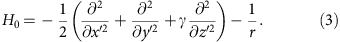The primed coordinates represent the laboratory frame, and we make a coordinate transformation,37 so that the kinetic energy operator is isotropic (and consequently the Coulomb potential is now anisotropic):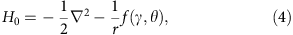where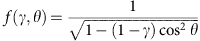and θ is the polar zenith angle (that is, angle down from the z axis) in the transformed frame. We write the wavefunction as a series of functions in separated polar coordinateswhere YL,m are the spherical harmonics. The UL,m are the wavefunctions to be found. Using the YL,m as the basis, the Schrödinger Equation using the Hamiltonian of equation 4 in matrix form iswhere E is the energy and. FL,m,L′,m′ is zero unless m=m′ and L has the same parity as L′, so equation 5 produces independent series of levels according to m and parity of L.

The values of FL,m,L′,m′ were found by numerically calculating the overlap integral of the spherical harmonic functions, and the secular equation was set up using the finite element approximation to the second derivative. To find the lowest energy-bound states for any given parity and m, this large matrix diagonalization problem was solved using the Lanczos numerical procedure, encoded in ARPACK library routines for Fortran.

In silicon donors, we choose z to be along the valley axis, and in general, the field in the laboratory frame, B′, can now have components in the xy plane. We choose the symmetric gauge1, AB × r, and for donors the apparent magnetic field in the transformed frame is36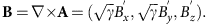The linear Zeeman Hamiltonian is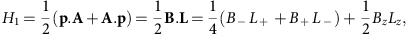where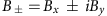, L is the angular momentum andare the raising and lowering operators. The quadratic Zeeman Hamiltonian is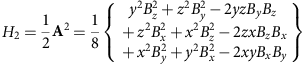in which we substituted the Cartesian definitions of the spherical harmonics.

The matrix elements of Lz lead to an additional diagonal term in equation 5, and is the main contribution to the Zeeman effect at small field along z, and those of Y0,0 lead to diagonal terms quadratic in field (for all field directions). The matrix elements of L±, which appear for non-zero Bxy, couple states with Δm=±1, and ΔL=0. The matrix elements of Y2,m between the basis states are given by Gaunt Coefficients that we evaluated analytically using Wigner 3j symbols. The Y2,0 term couples states with Δm=0 and ΔL=0,±2 and appears for all field directions (except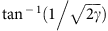to the crystal axis); Y2,±1 couples states with Δm=±1 and ΔL=0,±2 and appears when B is neither parallel nor perpendicular to z; and Y2,±2 couples states with Δm=±2 and ΔL=0,±2 and appears when there is a component of field in the xy plane.

### The Zeeman effect in hydrogen and silicon donors with B||z

For free hydrogen atoms, or for B′ along  for silicon donors, we are left only with Lz terms in H1 and Y0,0 and Y2,0 terms in H2. Of these, only Y2,0 produces off-diagonal terms. The non-zero off-diagonal matrix elements allow coupling by the magnetic field of spherical harmonic basis states with Δm=0 and ΔL=0,±2. This is the same set that is coupled by f in the case of donors in silicon, so the donor and hydrogen problems are therefore of the same complexity and can be separated for each different value of m and L parity independently. We solved it using a Lanczos procedure just as for donors in zero field. Using the spherical harmonics up to L=15 as a basis was sufficient for the Lyman series, but L=30 was found to be necessary for the Balmer series. The Lyman calculations of Figs 2, 3 took a few tens of minutes on a standard laptop. Convergence tests show that the results are accurate to better than 1 part in 104 for the highest energy transitions shown.

### The Zeeman effect in silicon donors with B′x,y≠0

For Bxy≠0, H1 includes L± terms and H2 includes Y2,±1 and Y2,±2 terms, which break the Δm=0 selection rule. This leaves only parity of L as a good quantum number, that is, we now have to include in the basis all spherical harmonics of a given L parity in the basis. This greatly increases the complexity of the problem. We used the Lanczos procedure to solve the coupling due to the Lz and Y2,0 terms only, and treated the remaining terms as a perturbation in the new basis of the new wavefunctions. In doing so we included the 10 states with lowest energy for each L parity and m up to m=±6 and we then diagonalised the Y2,±1 and Y2,±2 interactions between these states exactly.

### Transition rates

The electric dipole approximation for the transition rate caused by an oscillating electric field ɛ contains the matrix element (in the laboratory frame) of ɛ .r′ where the field in the transformed frame is. In terms of the spherical harmonics: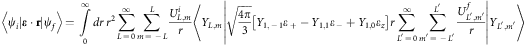This contains a sum of Gaunt coefficients, each related to a different light polarization. Note that these transitions involve states with opposite L parity. The calculation therefore requires calculation of both sets of parity states. In the case of B||z, the m states are not mixed, so for the Lyman series (Figs 2,3) we only need −1<m<1, for the Balmer series (Fig. 1) we need −2<m<2 and so on. Figure 2c shows the experimental verification of the selection rules, that is, the reversal of the field direction reverses the sense of circular polarization needed.

How to cite this article: Murdin, B.N. et al. Si:P as a laboratory analogue for hydrogen on high magnetic field white dwarf stars. Nat. Commun. 4:1469 doi: 10.1038/ncomms2466 (2013).

## References

1. Garstang, R. H. . Atoms in high magnetic-fields. Rep. Prog. Phys. 40, 105–154 (1977).

2. Ruder, H., Wunner, G., Herold, H. & Geyer, F. . Atoms in Strong Magnetic Fields: Quantum Mechanical Treatment And Applications In Astrophysics And Quantum Chaos Springer-Verlag (1994).

3. Schmelcher, P. & Schweizer, W. . Atoms And Molecules In Strong External Fields eBook edn Kluwer Academic Publisher (2002).

4. Cederbaum, L. S., Kulander, K. C. & March, N. H. . Atoms And Molecules In Intense Fields Springer-Verlag (1997).

5. Wickramasinghe, D. T. & Ferrario, L. . Magnetism in isolated and binary white dwarfs. Publ. Astron. Soc. Pac. 112, 873–924 (2000).

6. Vennes, S. et al. A multiwavelength study of the high-field magnetic white dwarf EUVE J0317-85.5 (=RE J0317-853). Astrophys. J. 593, 1040–1048 (2003).

7. Vanlandingham, K. M. et al. Magnetic white dwarfs from the SDSS. II. The second and third data releases. Astron. J. 130, 734–741 (2005).

8. Nordhaus, J. et al. Formation of high-field magnetic white dwarfs from common envelopes. Proc. Natl Acad. Sci. USA 108, 3135–3140 (2011).

9. Schmidt, G. D. & Norsworthy, J. E. . Rotation and magnetism in white-dwarfs. Astrophys. J. 366, 270–276 (1991).

10. Becken, W., Schmelcher, P. & Diakonos, F. K. . The helium atom in a strong magnetic field. J. Phys. B At. Mol. Opt. Phys. 32, 1557–1584 (1999).

11. Becken, W. & Schmelcher, P. . Non-zero angular momentum states of the helium atom in a strong magnetic field. J. Phys. B At. Mol. Opt. Phys. 33, 545–568 (2000).

12. Becken, W. & Schmelcher, P. . Higher-angular-momentum states of the helium atom in a strong magnetic field. Phys. Rev. A 63, 053412 (2001).

13. Schiff, L. I. & Snyder, H. . Theory of the quadratic Zeeman Effect. Phys. Rev. 55, 59–63 (1939).

14. Holle, A. et al. Diamagnetism of the hydrogen atom in the quasi-Landau regime. Phys. Rev. Lett. 56, 2594–2597 (1986).

15. Droungas, G., Karapanagioti, N. E. & Connerade, J. P. . Barium spectrum in high magnetic fields. Phys. Rev. A 51, 191–196 (1995).

16. Zhou, W. et al. Magnetic field control of the quantum chaotic dynamics of hydrogen analogs in an anisotropic crystal field. Phys. Rev. Lett. 105, 024101 (2010).

17. Friedrich, H. & Wintgen, D. . The hydrogen-atom in a uniform magnetic-field—an example of chaos. Phys. Rep. Rev. Sec. Phys. Lett. 183, 37–79 (1989).

18. Holle, A. et al. Hydrogenic rydberg atoms in strong magnetic-fields—theoretical and experimental spectra in the transition region from regularity to irregularity. Z. Phys. D Atoms Mol. Clusters 5, 279–285 (1987).

19. Lange, K. K., Tellgren, E. I., Hoffmann, M. R. & Helgaker, T. . A paramagnetic bonding mechanism for diatomics in strong magnetic fields. Science 337, 327–331 (2012).

20. Avron, J. E., Herbst, I. W. & Simon, B. . Separation of center of mass in homogeneous magnetic-fields. Ann. Phys. 114, 431–451 (1978).

21. Dippel, O., Schmelcher, P. & Cederbaum, L. S. . Charged anisotropic harmonic oscillator and the hydrogen atom in crossed fields. Phys. Rev. A 49, 4415–4429 (1994).

22. Motokawa, M. . Physics in high magnetic fields. Rep. Prog. Phys. 67, 1995–2052 (2004).

23. Pettinari, G. et al. Compositional evolution of bi-induced acceptor states in GaA1-xBix alloy. Phys. Rev. B 83, 201201 (2011).

24. Detmer, T., Schmelcher, P., Diakonos, F. K. & Cederbaum, L. S. . Hydrogen molecule in magnetic fields: The ground states of the sigma manifold of the parallel configuration. Phys. Rev. A 56, 1825–1838 (1997).

25. Detmer, T., Schmelcher, P. & Cederbaum, L. S. . Hydrogen molecule in a magnetic field: The lowest states of the pi manifold and the global ground state of the parallel configuration. Phys. Rev. A 57, 1767–1777 (1998).

26. Schmelcher, P., Detmer, T. & Cederbaum, L. S. . Excited states of the hydrogen molecule in magnetic fields: the singlet sigma states of the parallel configuration. Phys. Rev. A 61, 043411 (2000).

27. Schmelcher, P., Detmer, T. & Cederbaum, L. S. . Excited states of the hydrogen molecule in magnetic fields: the triplet sigma states of the parallel configuration. Phys. Rev. A 64, 023410 (2001).

28. Ramdas, A. K. & Rodriguez, S. . Spectroscopy of the solid-state analogs of the hydrogen-atom—donors and acceptors in semiconductors. Rep. Prog. Phys. 44, 1297–1387 (1981).

29. Steger, M. et al. Shallow impurity absorption spectroscopy in isotopically enriched silicon. Phys. Rev. B 79, 205210 (2009).

30. Vinh, N. Q. et al. Silicon as a model ion trap: Time domain measurements of donor rydberg states. Proc. Natl Acad. Sci. USA 105, 10649–10653 (2008).

31. Greenland, P. T. et al. Coherent control of rydberg states in silicon. Nature 465, 1057–1061 (2010).

32. Simmons, S. et al. Entanglement in a solid-state spin ensemble. Nature 470, 69–72 (2011).

33. Zawadzki, W., Song, X. N., Littler, C. L. & Seiler, D. G. . High excited states of magnetodonors in InSb: An experimental and theoretical study. Phys. Rev. B 42, 5260–5269 (1990).

34. Bimberg, D. . Anomaly of the linear and quadratic Zeeman effect of an effective-mass acceptor: C in GaAs. Phys. Rev. B 18, 1794–1799 (1978).

35. Barmby, P. W., Dunn, J. L., Bates, C. A. & Klaassen, T. O. . High-field calculations of Landau-like shallow donor states: A finite-difference approach. Phys. Rev. B 57, 9682–9689 (1998).

36. Grossmann, G., Bergman, K. & Kleverman, M. . Spectroscopic studies of double donors in silicon. Physica B+C 146, 30–46 (1987).

37. Thilderkvist, A., Kleverman, M., Grossmann, G. & Grimmeiss, H. G. . Quadratic Zeeman effect of shallow donors in silicon. Phys. Rev. B 49, 14270–14281 (1994).

38. Bruno-Alfonso, A., Lewis, R. A., Hai, G. Q. & Vickers, R. E. M. . Energy states of phosphorous donor in silicon in fields up to 18 T. J. Low Temp. Phys. 159, 226–229 (2010).

## Acknowledgements

We are grateful for conversations with G. Aeppli and P.T. Greenland. We acknowledge financial support from the UK Engineering and Physical Sciences Research Council (grant references EP/H026622/1 ‘COMPASSS’, EP/H001905/1, EP/E061265/1). J.L. is grateful to EPSRC for a Doctoral Prize Fellowship. Part of this work has been supported by EuroMagNET II under EU Contract No. 228043.

## Author information

Authors

### Contributions

B.N.M. and C.R.P. designed the research programme; J.L., K.L.L., S.K.C., H.E., E.T.B. performed the high-field experiments; M.L.Y.P. did the computations; N.V.A., H.R. grew the crystal; S.G.P. and H.-W.H. analysed and prepared the crystal; and B.N.M., C.R.P., I.G. and P.G.M. led the analysis and wrote the paper.

### Corresponding author

Correspondence to B.N. Murdin.

## Ethics declarations

### Competing interests

The authors declare no competing financial interests.

## Supplementary information

### Supplementary Data 1

Data obtained from the sample 'Corretto', growth method Fz, doping concentration 8×1014 cm-3, with field step of 1T. (TXT 6037 kb)

### Supplementary Data 1

Data obtained from the sample 'Double Espresso', i.e. two 'Espresso' samples mounted together in a stack. The file contains only -30 and +30T interferograms. Sample growth method Cz, doping concentration 6-20×1014 cm-3. (TXT 35 kb)

### Supplementary Data 1

Data obtained from the sample 'Latte', growth method Cz, doping concentration 6-10×1014 cm-3, with field step of 0.25T. (TXT 2430 kb)

## Rights and permissions

Reprints and Permissions

Murdin, B., Li, J., Pang, M. et al. Si:P as a laboratory analogue for hydrogen on high magnetic field white dwarf stars. Nat Commun 4, 1469 (2013). https://doi.org/10.1038/ncomms2466

• Accepted:

• Published:

• DOI: https://doi.org/10.1038/ncomms2466

• ### The multi-photon induced Fano effect

• K. L. Litvinenko
• Nguyen H. Le
• B. N. Murdin

Nature Communications (2021)

• ### Giant non-linear susceptibility of hydrogenic donors in silicon and germanium

• Nguyen H. Le
• Grigory V. Lanskii
• Benedict N. Murdin

Light: Science & Applications (2019)

• ### Giant multiphoton absorption for THz resonances in silicon hydrogenic donors

• M. A. W. van Loon
• N. Stavrias
• B. N. Murdin

Nature Photonics (2018)

• ### Coherent superpositions of three states for phosphorous donors in silicon prepared using THz radiation

• S. Chick
• N. Stavrias
• B. N. Murdin

Nature Communications (2017)

• ### Coherent creation and destruction of orbital wavepackets in Si:P with electrical and optical read-out

• K.L. Litvinenko
• E.T. Bowyer
• B.N. Murdin

Nature Communications (2015)